# 978-0123869449 Chapter 3 Part 1

Document Type
Homework Help
Book Title
Authors
Michael F. Modest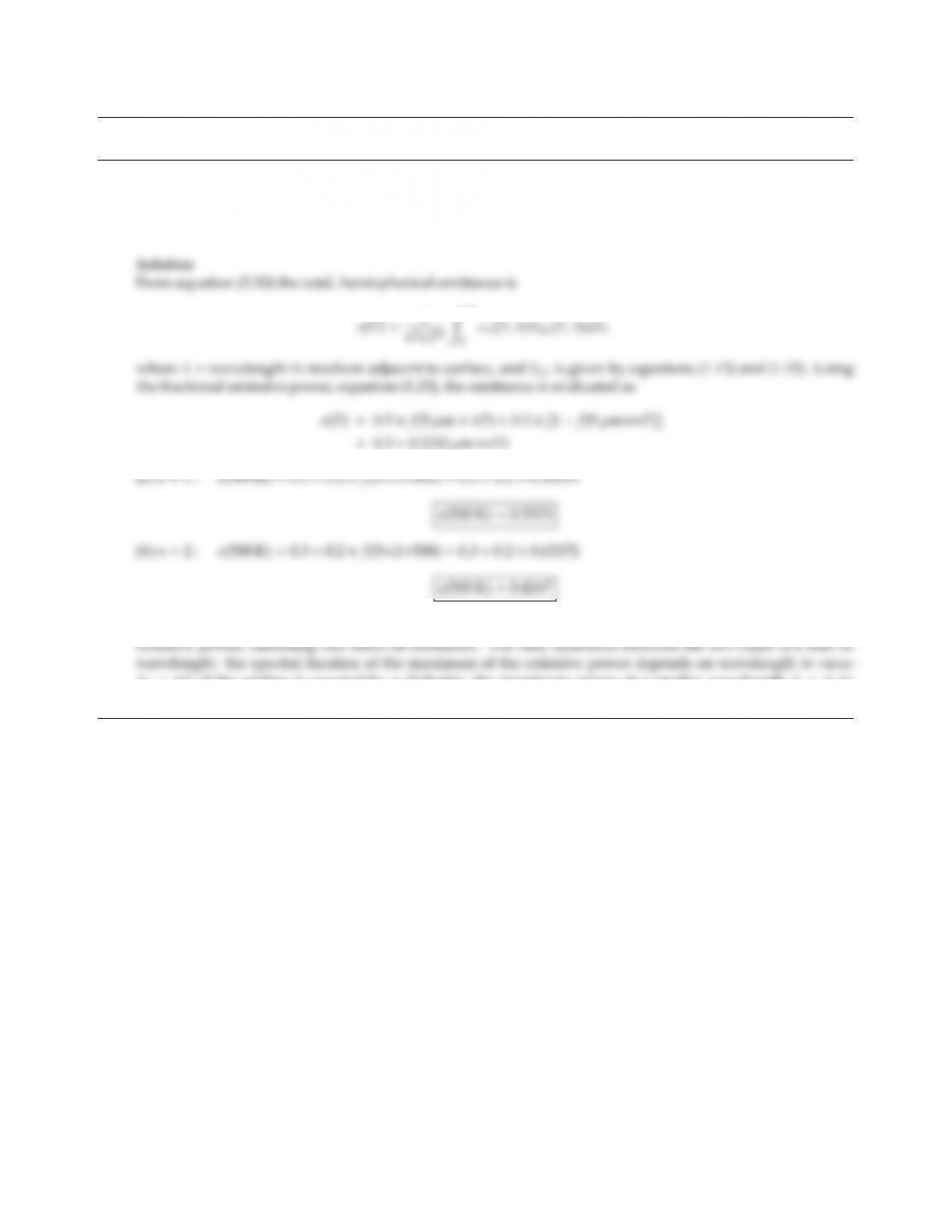CHAPTER 3
3.1 A diusely emitting surface at 500 K has a spectral, directional emittance that can be approximated by 0.5
in the range 0 < λ < 5µm and 0.3 for λ > 5µm. What is the total, hemispherical emittance of this surface
surrounded by (a) air and (b) a dielectric medium of refractive index n=2?
Note: while total emissive power is proportional to n2, this is true for both, the actual and the blackbody
λ0=nλ; if the surface is covered by a dielectric, the maximum occurs at a smaller wavelength λ=λ0/n
(where the present material has a higher emittance).
27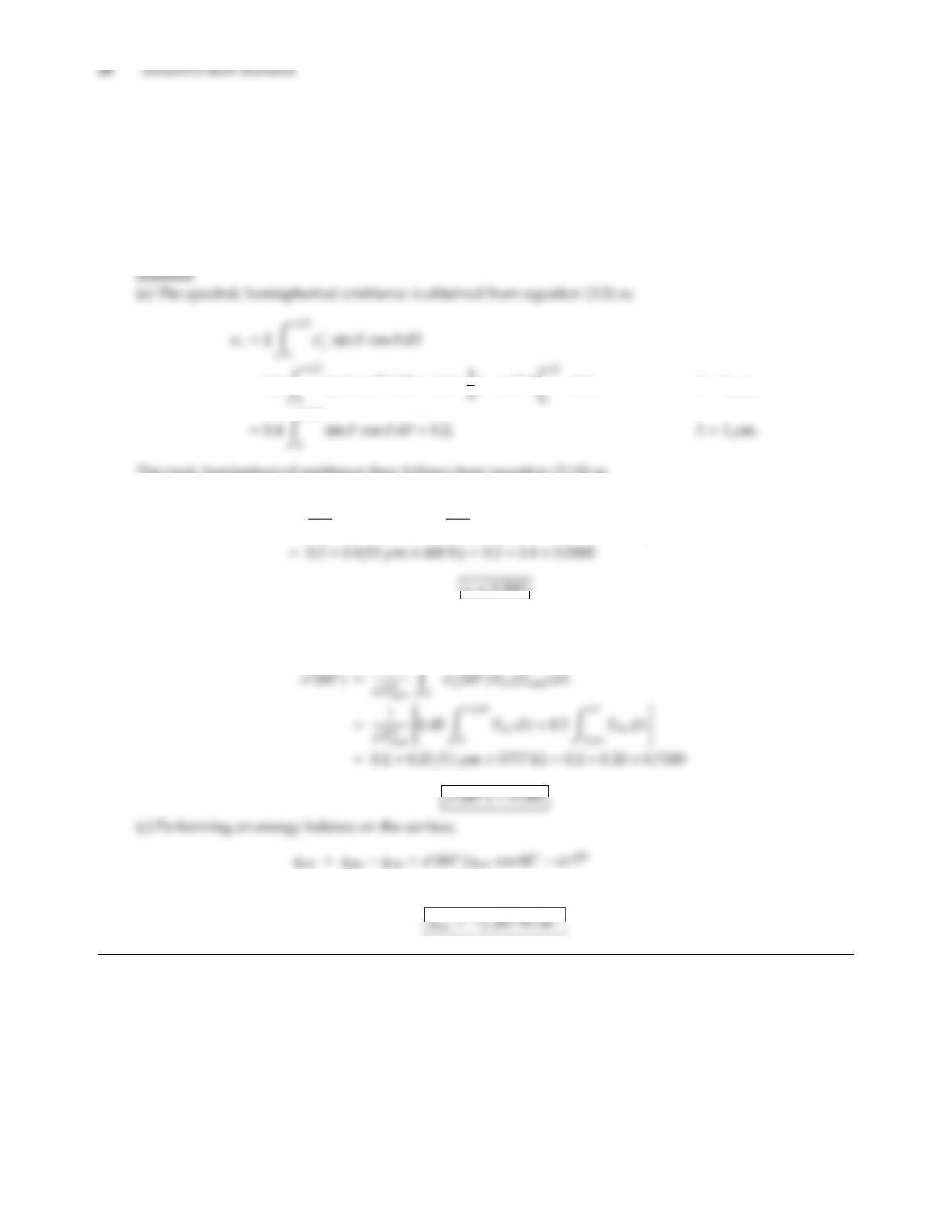3.2 A certain material at 600 K has the following spectral, directional emittance:
ǫ
λ=0.9 cos θ, λ < 1µm,
0.2, λ > 1µm,
(a) What is the total, hemispherical emittance of the material?
(b) If the sun irradiates this surface at an angle of θ=60o-normal, what is the relevant total absorptance?
(c) What is the net radiative energy gain or loss of this surface (per unit time and area)?
=1.8Zπ/2
sin θcos2θdθ=1.8×1
=0.6, λ < 1µm,
The total, hemispherical emittance then follows from equation (3.10) as
ǫ=1
σT4Z
0
ǫλEbλdλ=1
σT4"0.6Z1µm
0
Ebλdλ+0.2Z
1µm
Ebλdλ#
ǫ=0.200
(b) The relevant absorptance is the total, directional emittance at the solar incidence angle. From equa-
tions (3.15) and (3.23), and assuming IsunEbλ(Tsun),
=0.380 ×1367 W/m2×0.50.200 ×5.670 ×108×6004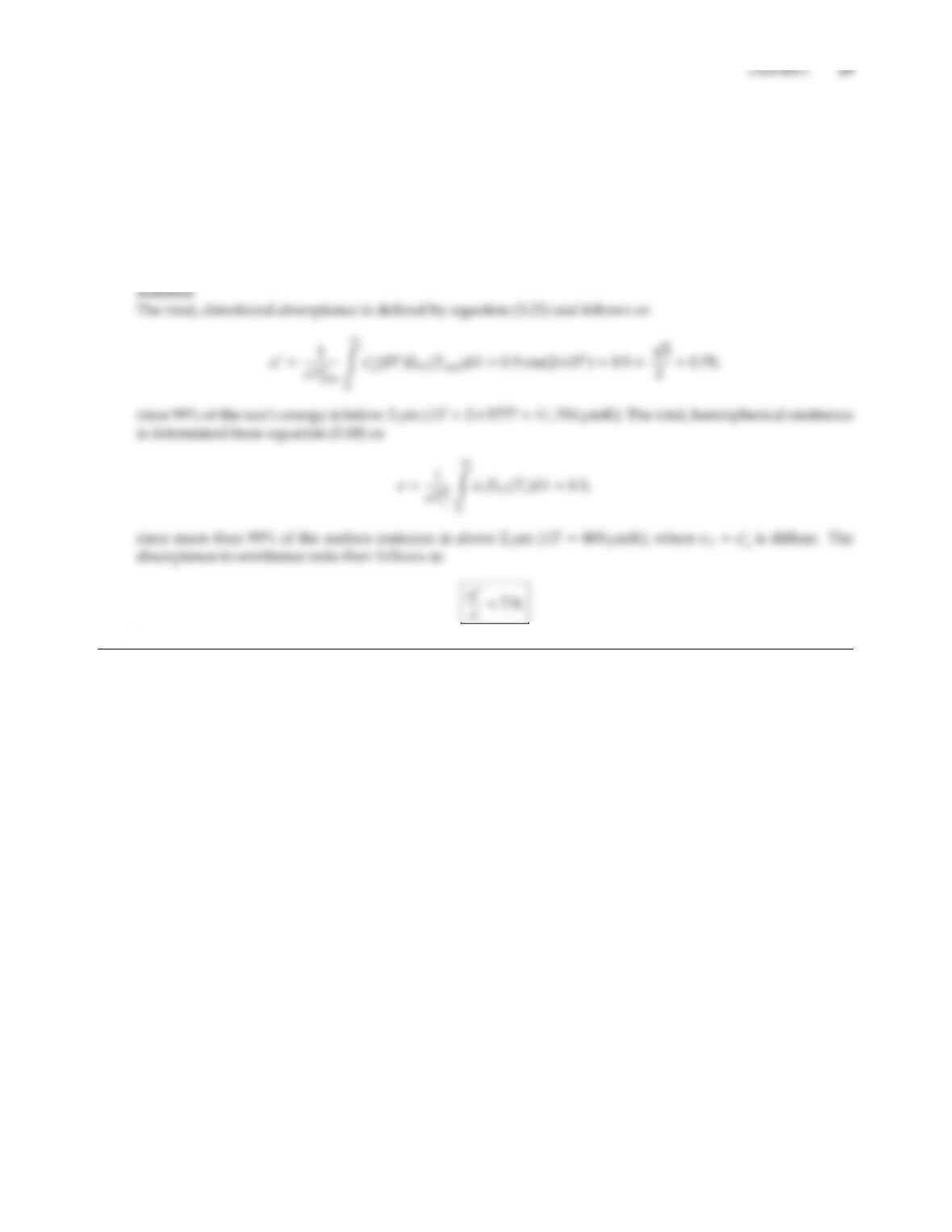3.3 For optimum performance a solar collector surface has been treated so that, for the spectral, directional
emittance:
ǫ
λ=(0.9 cos 2θ, θ < 45
0.0θ > 45), λ < 2µm,
=0.1,all θ, λ > 2µm.
For solar incidence of 15o-normal and a collector temperature of 400 K, what is the relevant ratio of
absorptance-to-emittance?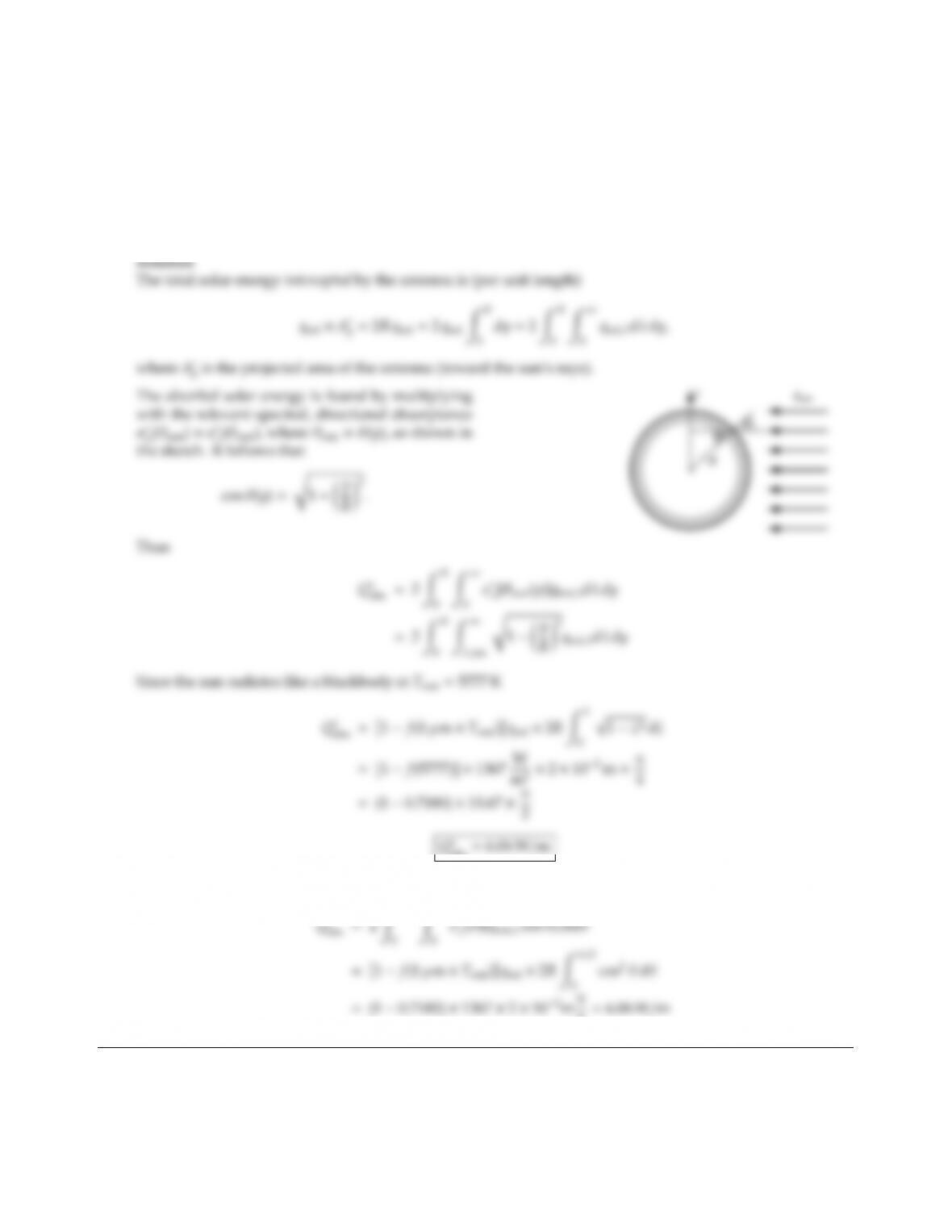3.4 A long, cylindrical antenna of 1 cm radius on an earth-orbiting satellite is coated with a material whose
emittance is
ǫ
λ=0, λ < 1µm,
cos θ, λ 1µm.
Find the absorbed energy per meter length. (Assume irradiation is from the sun only, and in a direction
normal to the antenna’s axis; neglect the earth and stars.)
abs =6.06 W/m
Alternatively, integrating over the surface area of the cylinder,
4=6.06 W/mCHAPTER 3 31
3.5 The spectral, hemispherical emittance of a (hypothetical) metal may be approximated by the relationship
ǫλ=0.5, λ < λc=0.5µm,
0.5λc/λ, λ > λc,
(independent of temperature). Determine the total, hemispherical emittance of this material using (a) Planck’s
law, (b) Wien’s distribution, for a surface temperature of (i) 300 K, (ii) 1000 K. How accurate is the prediction
using Wien’s distribution?thus, taking only the first terms in the φn: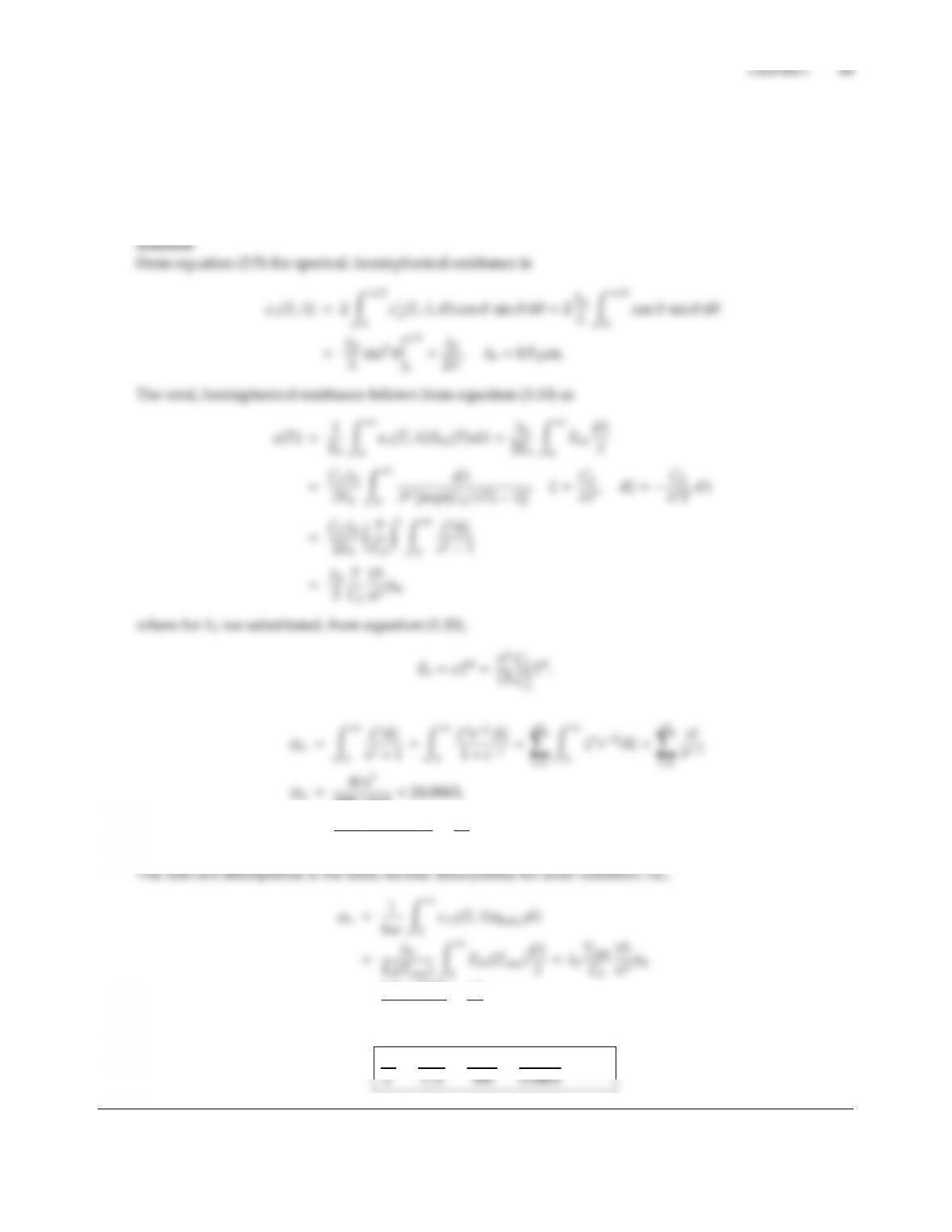3.6 A treated metallic surface is used as a solar collector material; its spectral, directional emittance may be
approximated by
ǫ
λ=0.5µm/λ, θ < 45,
0, θ > 45.
What is the relevant α/ǫ-ratio for near normal solar incidence if Tcoll 600 K?
295.1215 =24.8863,
ǫ(T)=0.5µm×600 K
2×14,388 µmK ×15
π4×24.8863 =0.0400.
=0.5×5777
14,388 ×15
π4×24.8863 =0.7694.
Thus
αn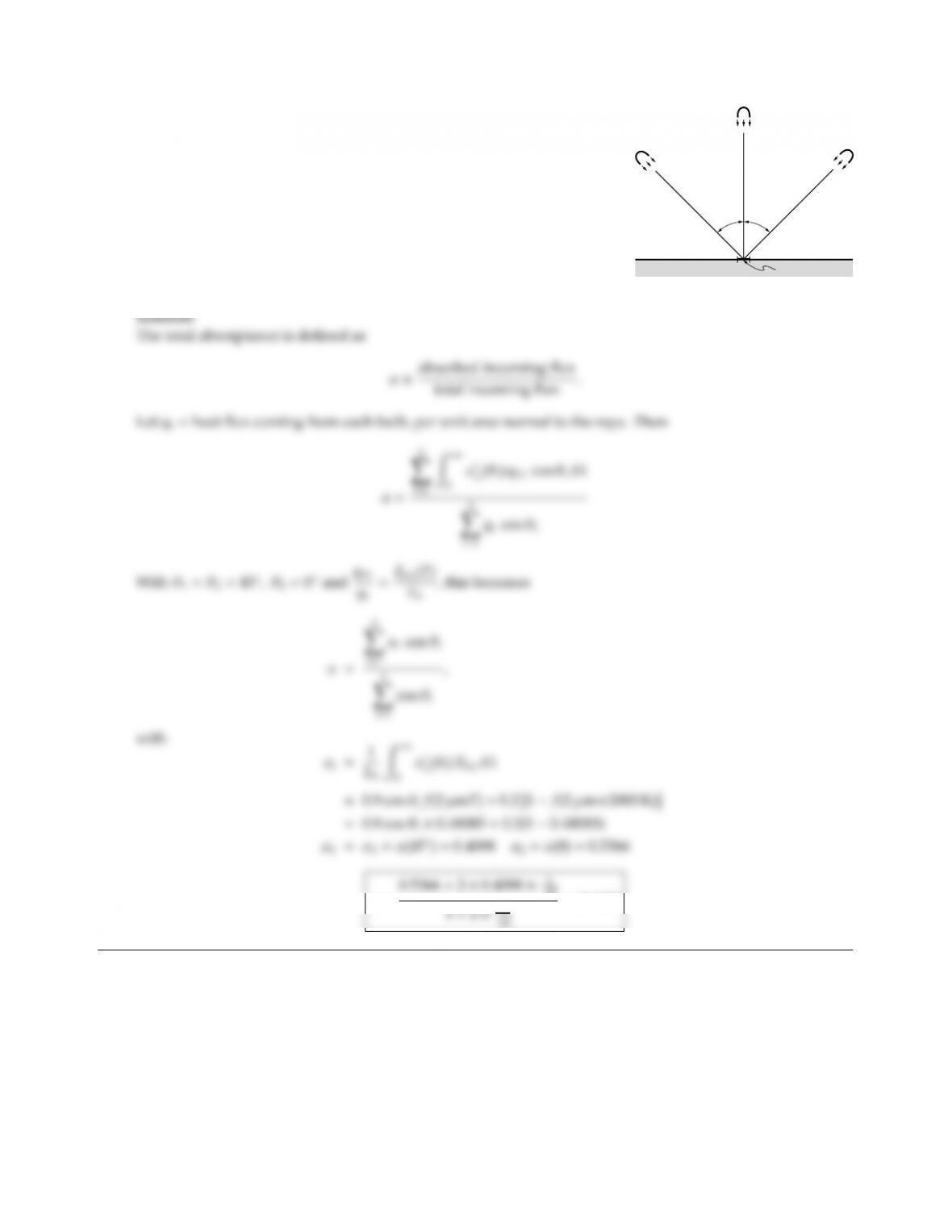3.7
Tungsten
lamps
45°45°
Sample
A surface sample with
ǫ
λ=0.9 cos θ, λ < 2µm,
0.2, λ > 2µm.
is irradiated by three tungsten lights as shown. The tungsten lights
may be approximated by black spheres at T=2000 K fitted with mir-
rors to produce parallel light beams aimed at the sample. Neglecting
background radiation, determine the absorptance of the sample.
α=
=0.4623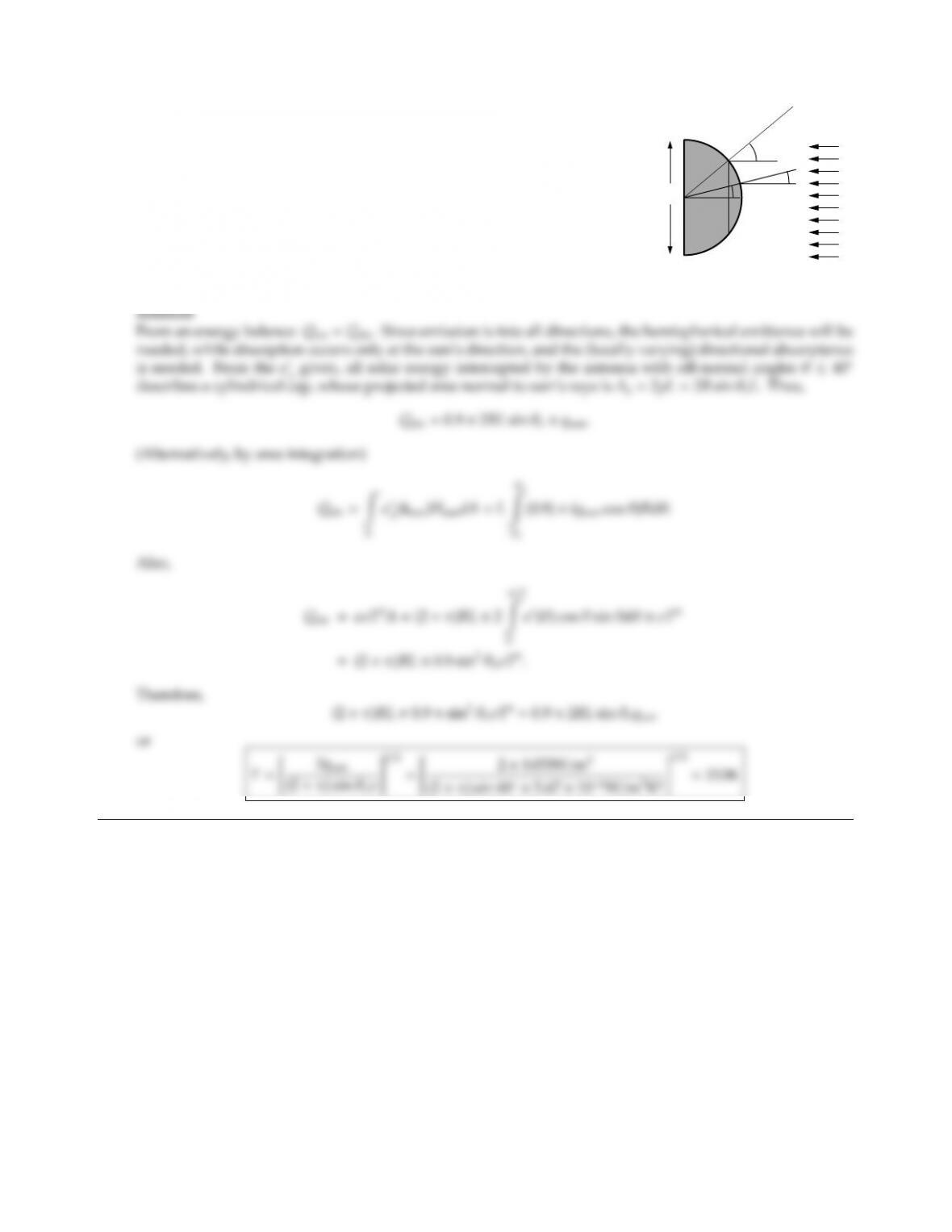CHAPTER 3 35
3.8
2R
θ
θ
qsun=1367W/m2
An antenna of a satellite may be approximated by a long half cylinder,
which is exposed to sunshine as shown in the sketch. The antenna has
a high conductivity (i.e., is isothermal), and is coated with the material
of Fig. 3-36, i.e., the material may assumed to be gray with the following
directional characteristics:
ǫ
λ=0.9,0θ < 40,
0, θ > 40.
Determine the equilibrium temperature of the antenna, assuming it ex-
changes heat only with the sun (and cold outer space).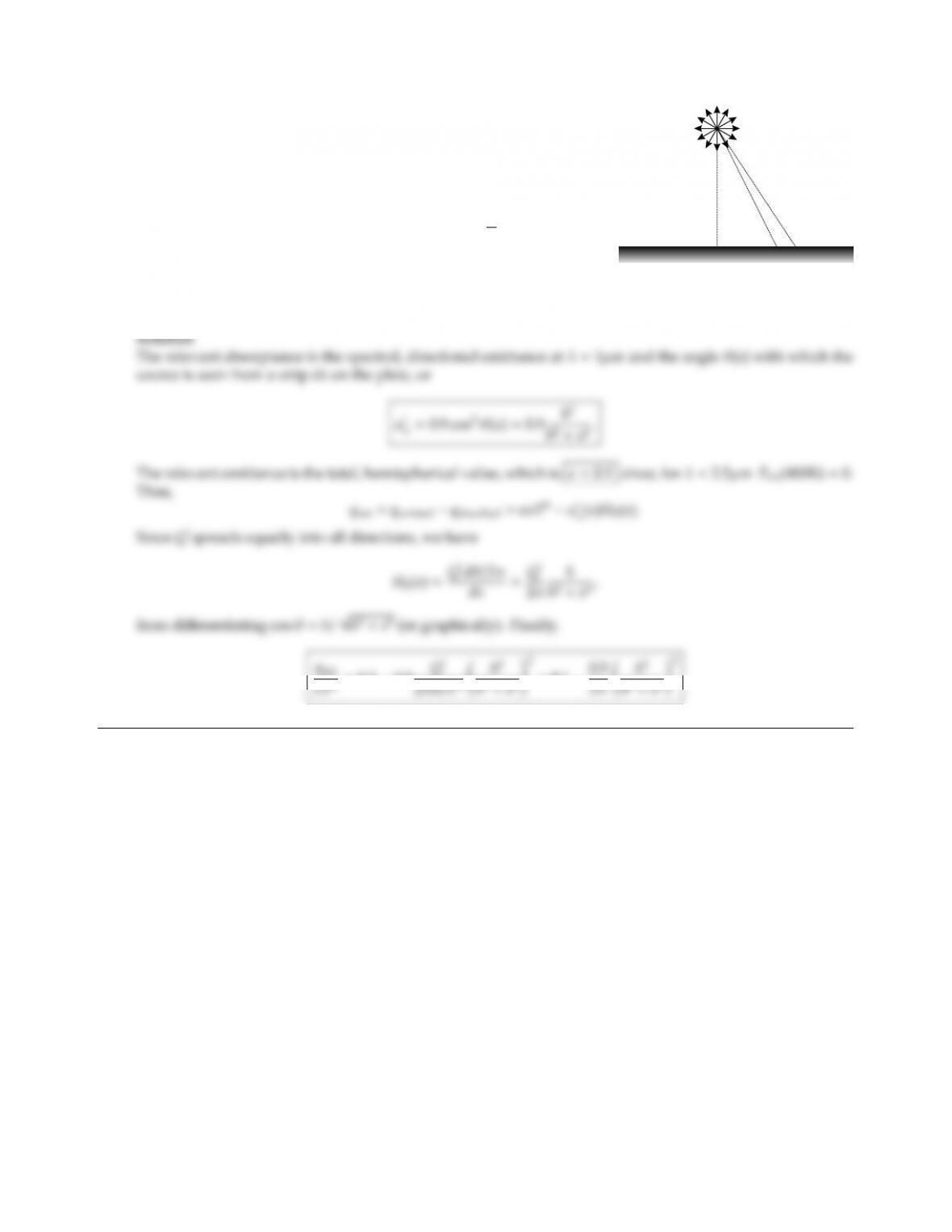3.9
h
dx
θ
Q'
A large isothermal plate (temperature T=400 K) is exposed to a
long monochromatic (λ=1µm) line source as shown. The strength
of the line source is Q(W/m length of source) =hσT4, spreading
equally into all directions. The plate has a spectral, directional
emittance of
ǫ
λ=(0.9 cos2θ,λ < 2.5µm,
0.1, λ > 2.5µm. 0θ < π
2
For a general location, x, determine relevant absorptance, emit-
tance, and the net local heat flux qnet(x), which must be supplied
to/removed from the plate to keep it isothermal at T.
=0.10.9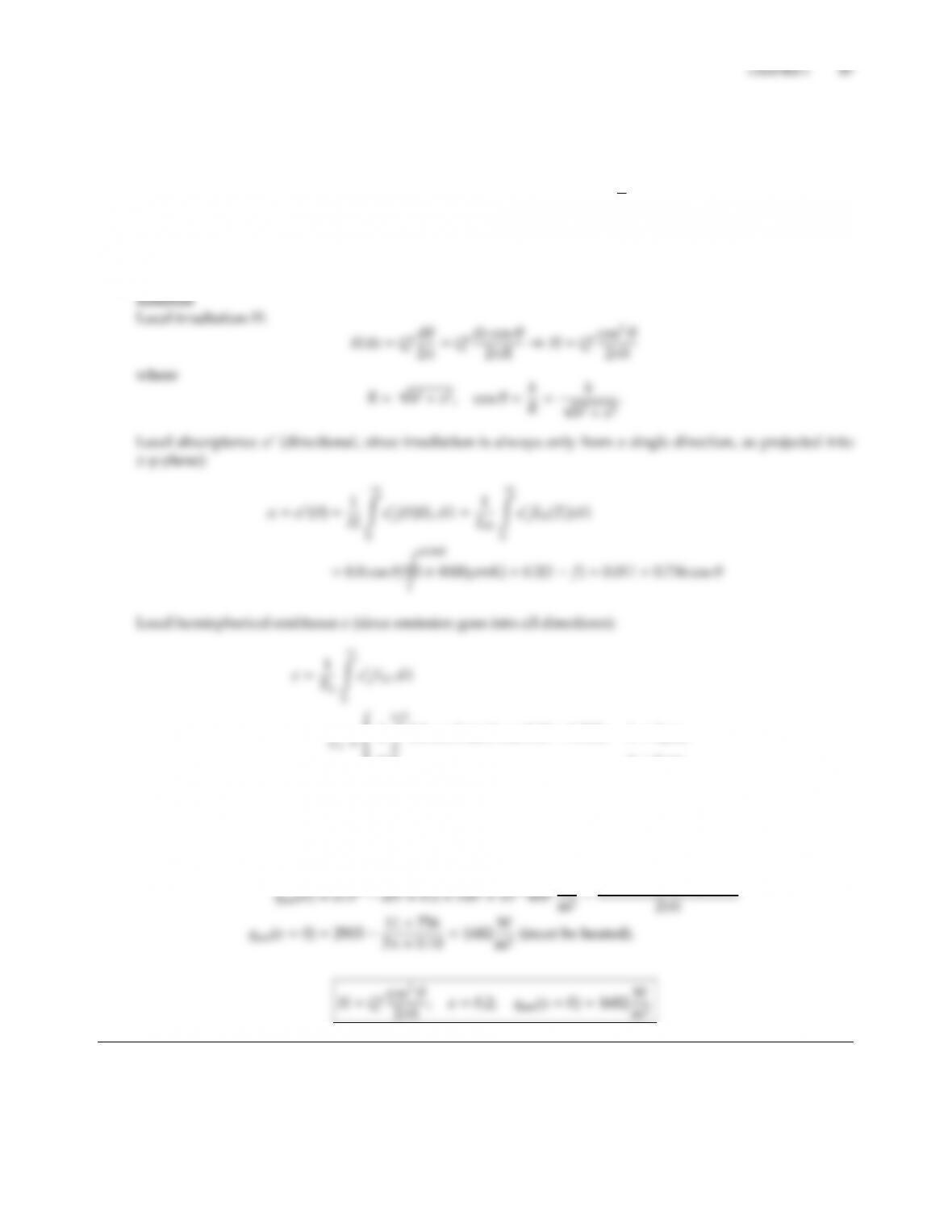3.10 A large isothermal plate (temperature T=400 K) is exposed to a long tungsten–halogen line source as shown
in the sketch next to Problem 3.9. The strength of the line source is Q=1000 W/m length of source, spreading
equally into all directions, and has the spectral distribution of a blackbody at 4000K. The plate has a spectral,
directional emittance of
ǫ
λ=(0.8 cos θ,λ < 3µm,
0.2, λ > 3µm. 0θ < π
2
For a general location, x, give an expression for local irradiation H, determine relevant absorptance, emittance,
and an expression for the net local heat flux qnet(x), which must be supplied to/removed from the plate to
keep it isothermal at T.
2
0.8 cos θsin θcos θdθ=0.533, λ < 3µm,
0.2, λ > 3µm.
ǫ=f,=0.002
(3 ×400µmK) +0.2(1 f)=0.2.
Local net flux: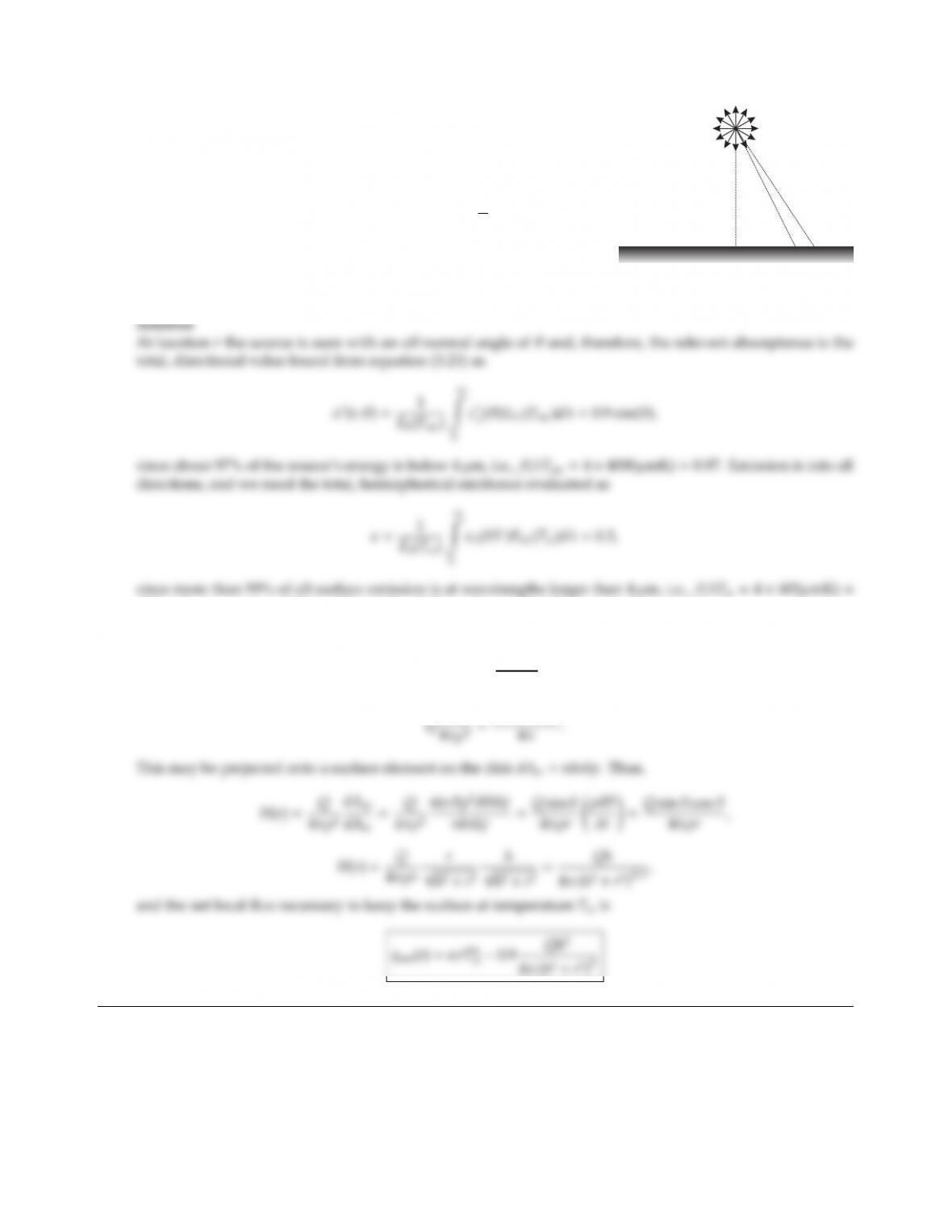3.11
h
dr
θ
Q
An isothermal disk (temperature T=400 K) is exposed to a small
black spherical source (temperature Ts=4000 K) as shown. The
strength of the source is Q(W), spreading equally into all directions.
The plate has a spectral, directional emittance of
ǫ
λ=(0.9 cos θ,λ < 4µm,
0.3, λ > 4µm. 0θ < π
2
For a general location, r, determine relevant absorptance, relevant
emittance, and the net local heat flux qnet(r), which must be supplied
to/removed from the plate to keep it isothermal at T.
0.01, where ǫλ=ǫ
λis diuse. The net flux follows from a simple energy balance as
qnet(r)=ǫσT4
wα(r)Ho(r).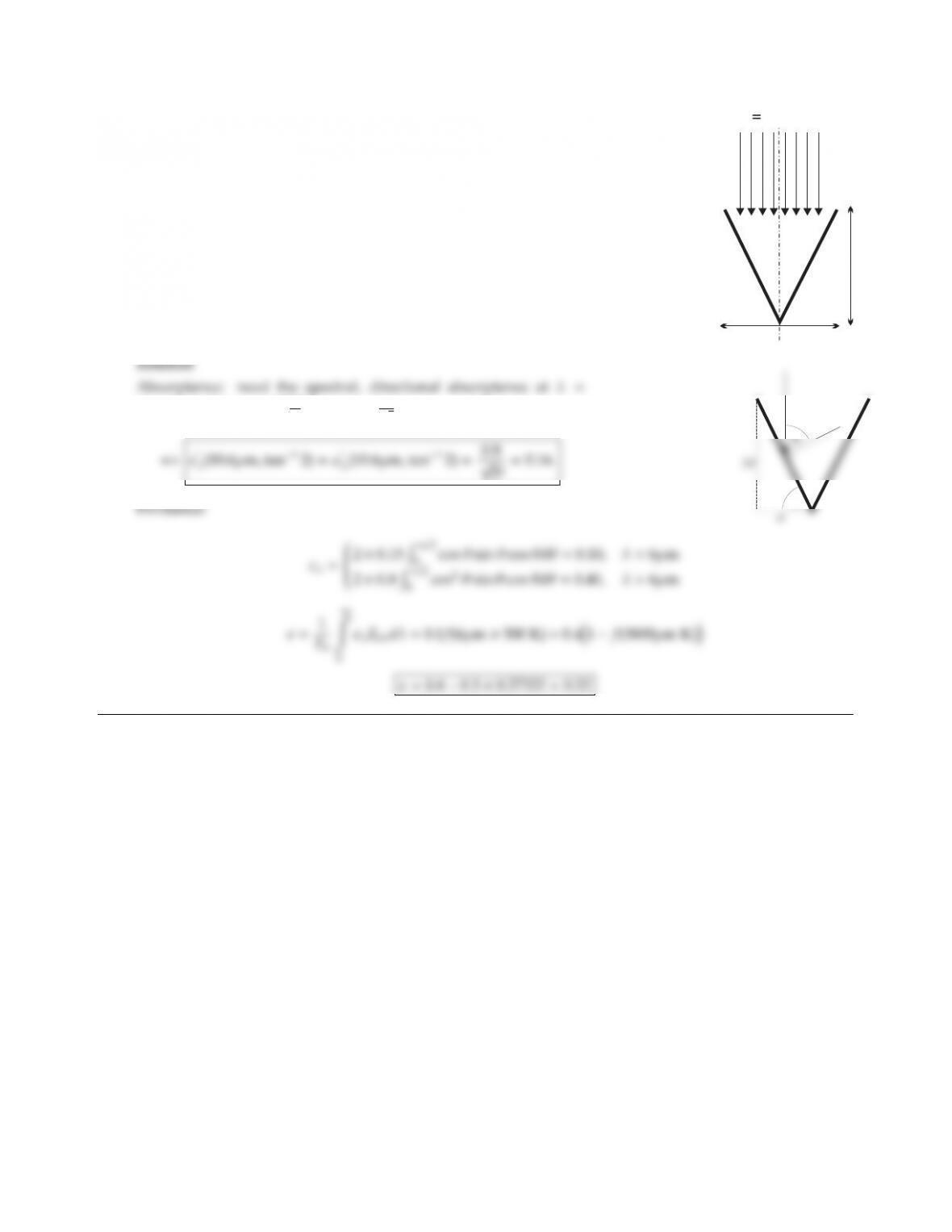CHAPTER 3 39
3.12
qL10 W/cm
3 2
2d
2d
A conical cavity is irradiated by a defocussed CO2laser (wavelength =10.6µm)
as shown. The conical surface is maintained at 500 K. For cavity coating with a
spectral, directional emittance
ǫ
λ(λ, θ)=0.15 cos θ, λ < 6µm,
0.8 cos2θ, λ > 6µm,
determine the relevant total absorptance and emittance.
10.6µm and θ=tan12d
dor cos θ=1
5
θ
i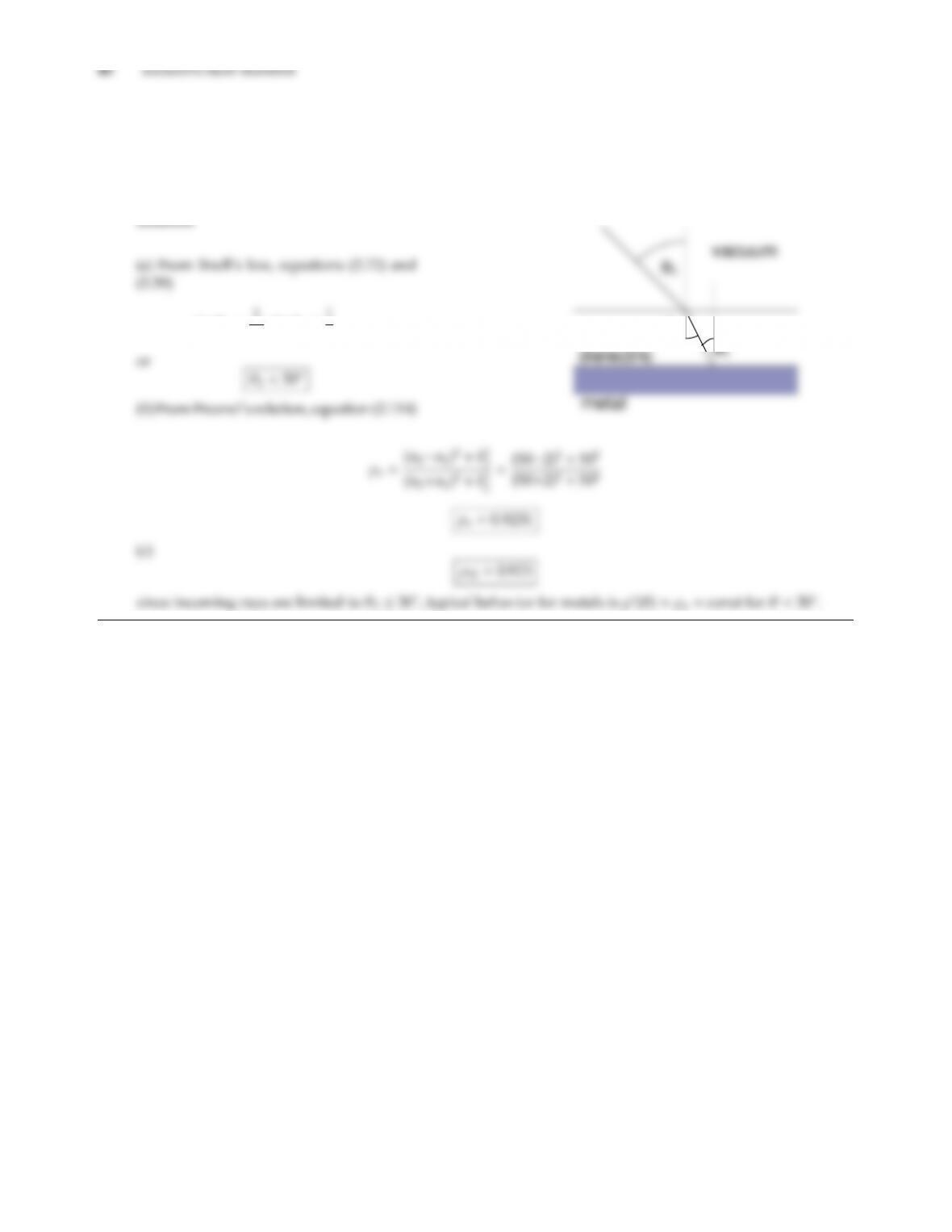3.13 A metal (m2=50 50 i) is coated with a dielectric (m1=20i), which is exposed to vacuum.
(a) What is the range of possible directions from which radiation can impinge on the metal?
(b) What is the normal reflectance of the dielectric-metal interface?
(c) What is the (approximate) relevant hemispherical reflectance for the dielectric-metal interface?
Solution
sin θ2=1
n1
sin θ11
2,
θ2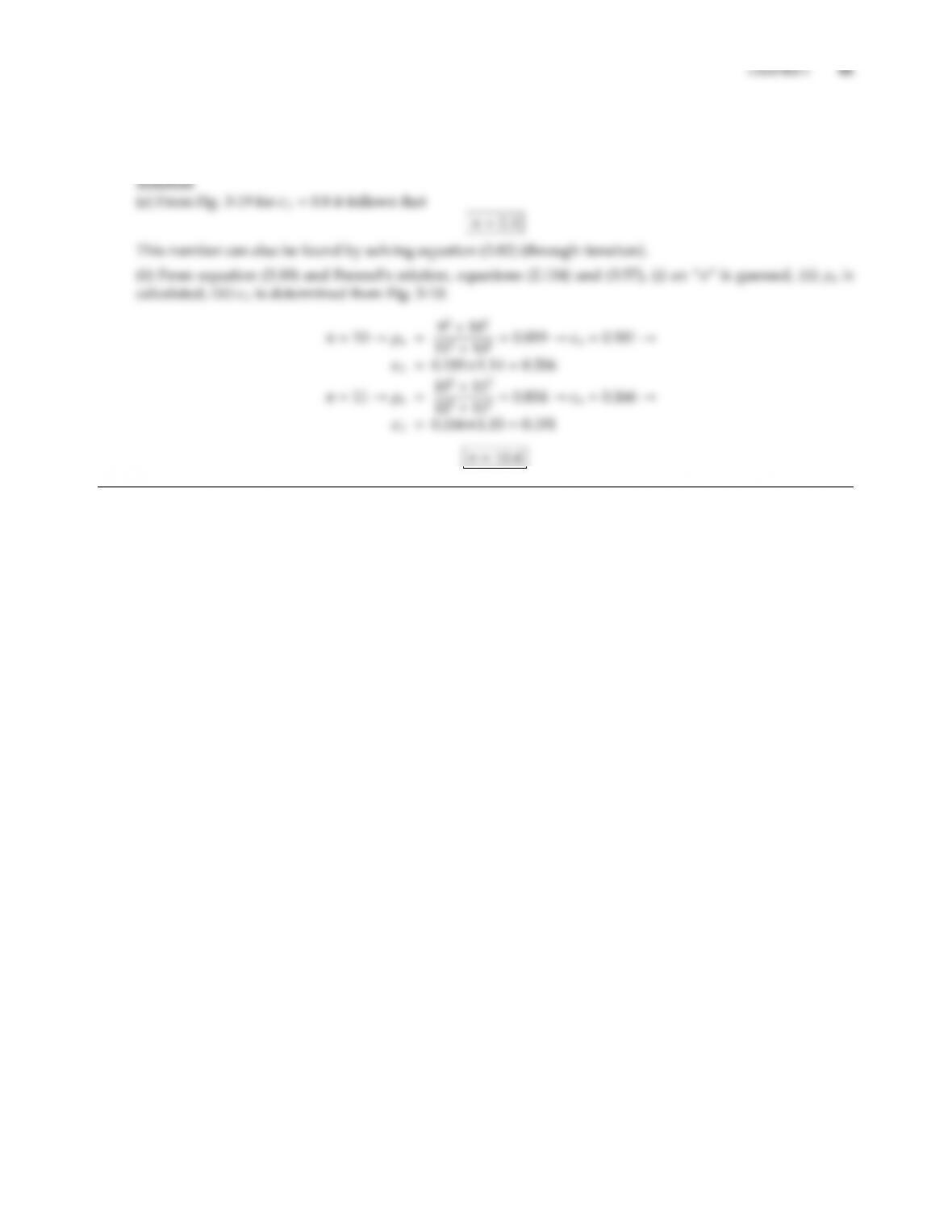3.14 For a certain material, temperature and wavelength the spectral, hemispherical emittance has been measured
as ǫλ. Estimate the refractive index of the material under these conditions, assuming the material to be (a) a
dielectric with ǫλ=0.8, (b) a metal in the infrared with ǫλ=0.2 (the Hagen-Rubens relation being valid).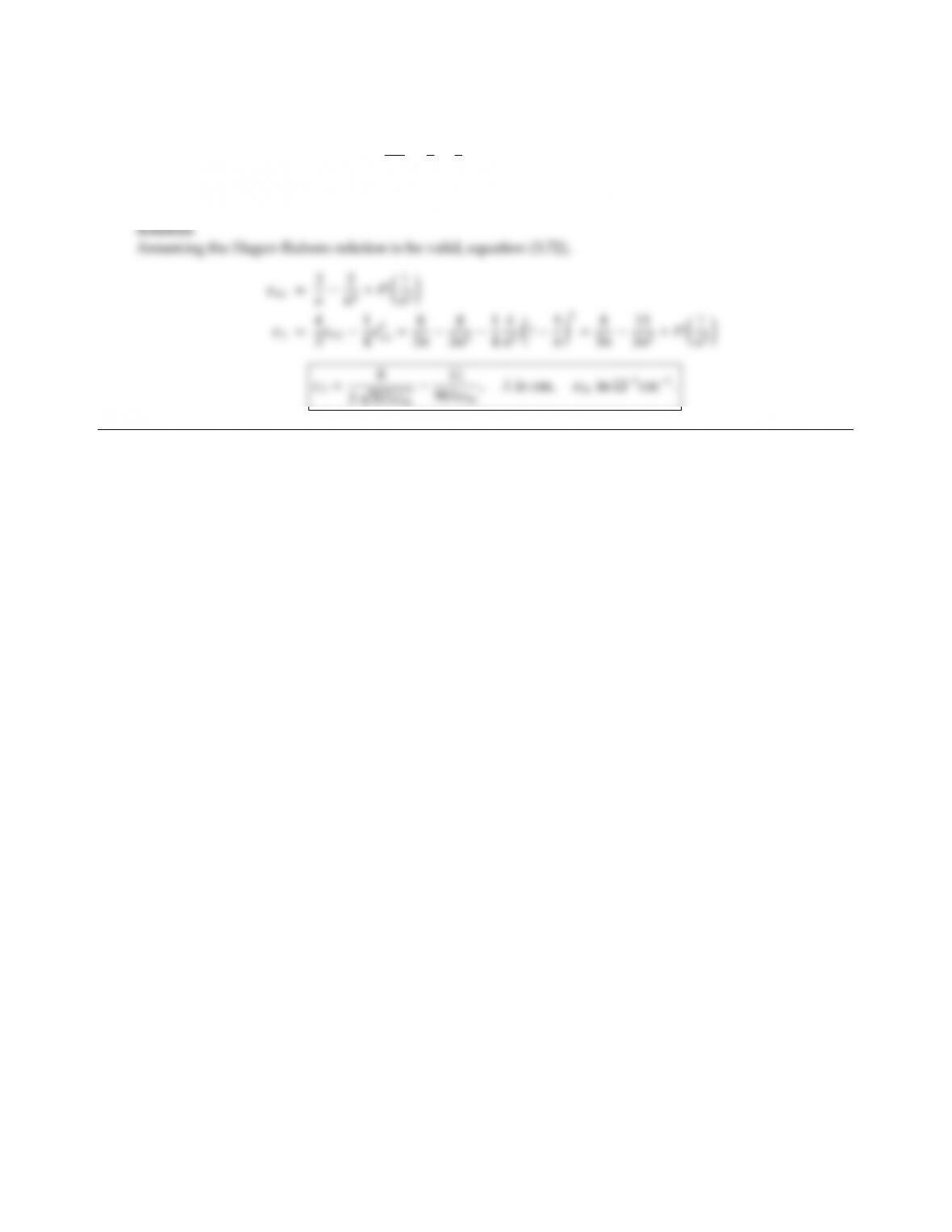3.15 It can be derived from electromagnetic wave theory that
ǫλ
ǫnλ4
31
4ǫnλfor ǫnλ1.
Determine ǫλfor metals with ǫnλ1 as a function of wavelength and temperature.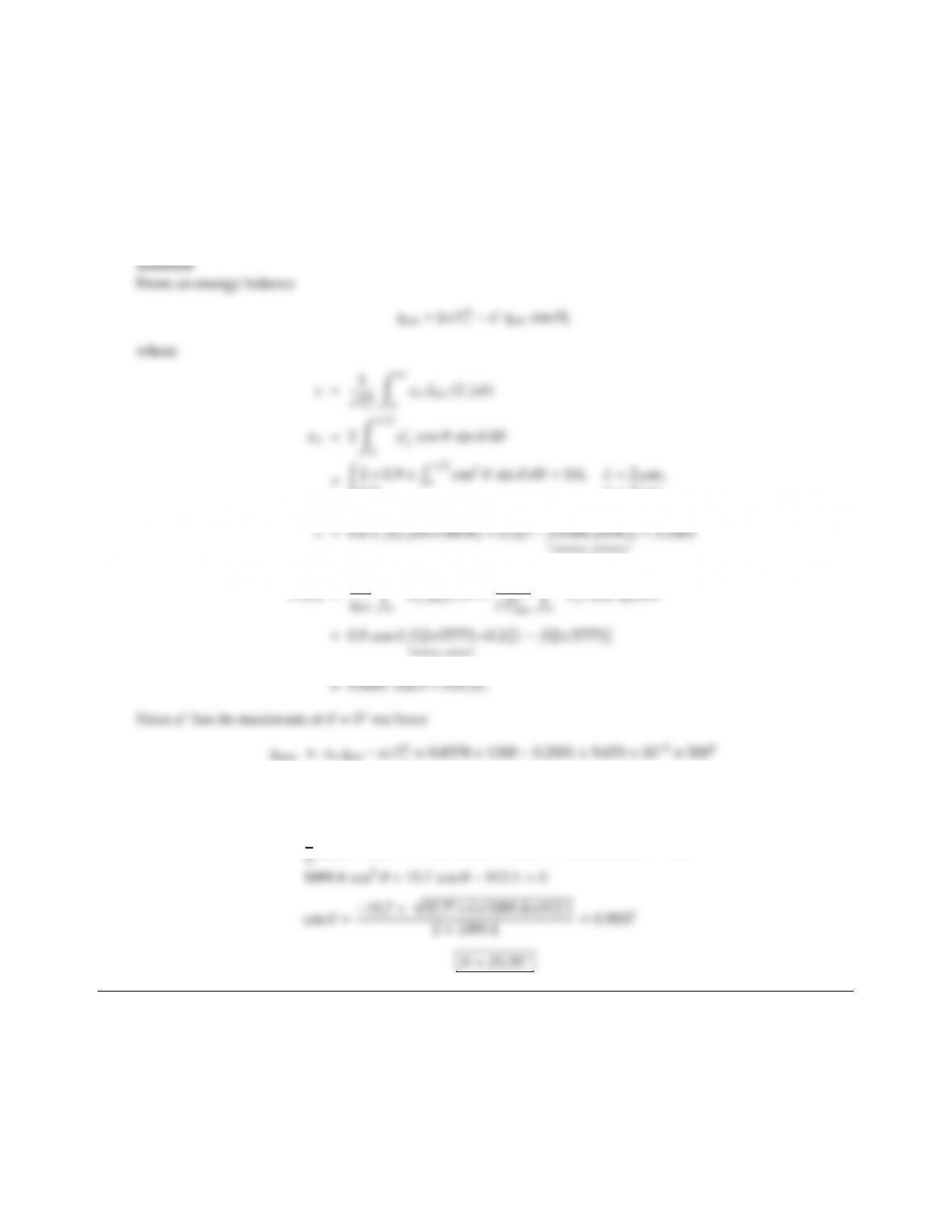CHAPTER 3 43
3.16 A solar collector surface with emittance
ǫ
λ=0.9 cos θ, λ < 2µm,
0.2, λ > 2µm.
is to be kept at Tc=500 K. For qsol =1300 W/m2, what is the range of possible sun positions with respect to
the surface for which at least 50% of the maximum net radiative energy is collected? Neglect conduction and
convection losses from the surface.
0.2, λ > 2µm,
and
0.003
α(θ)=1
α
ǫ
0.93962
=1115.1709.1=406.0 W/m2.
Half that maximum occurs at
1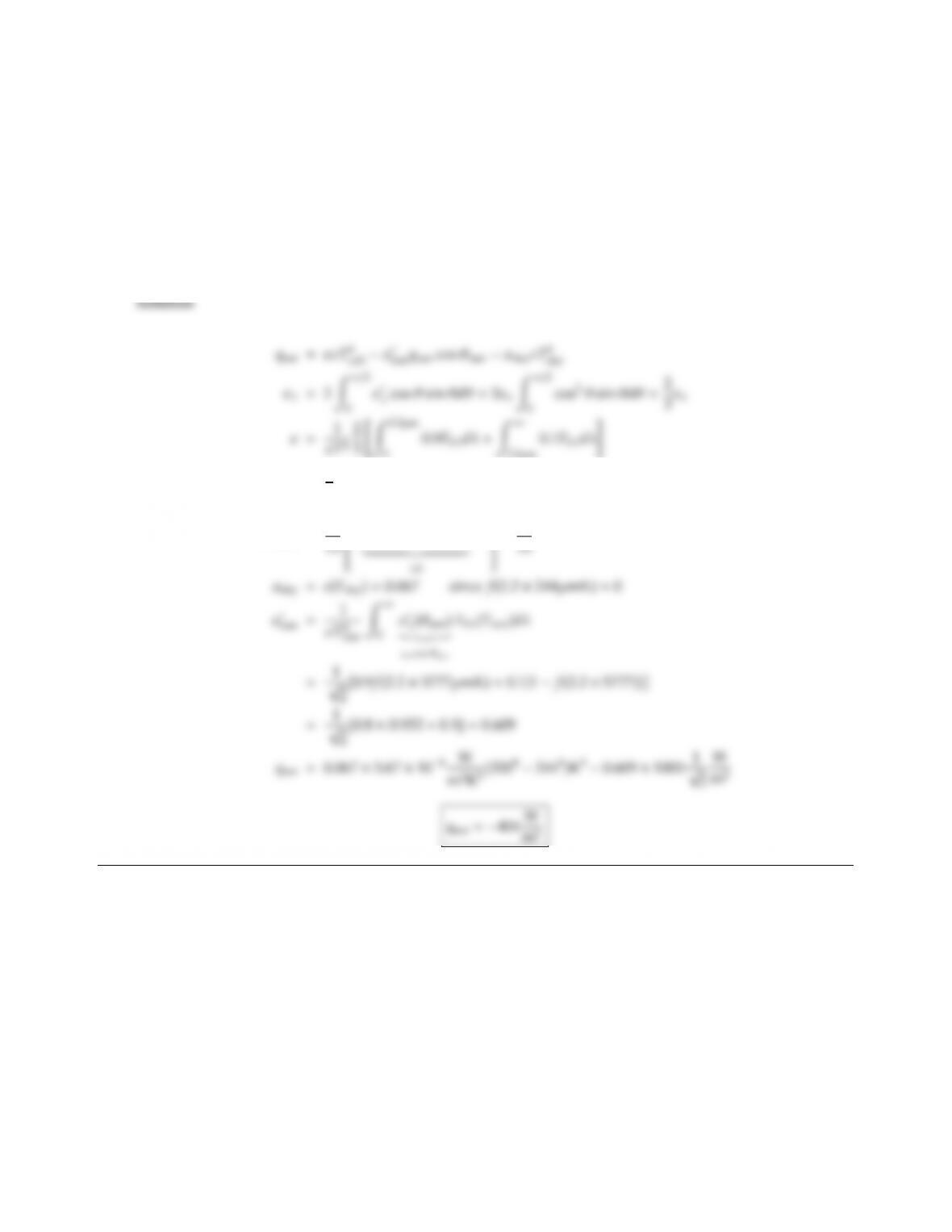3.17 On one of those famous clear days in Central Pennsylvania (home of PennState), a solar collector is irradiated
by direct sunshine and by a diuse atmospheric radiative flux. The magnitude of the solar flux is qsun =1000
W/m2(incident at θsun =45), and the eective blackbody temperature for the sky is Tsky =244 K. The
absorber plate is isothermal at 320 K and is covered with a nongray, nondiuse material whose spectral,
directional emittance may be approximated by
ǫ
λ(λ, θ)=ǫnλcos θ, ǫnλ=0.9, λ < 2.2µm,
0.1, λ > 2.2µm,
where ǫnλis the normal, spectral emittance. Determine the net radiative flux on the collector.
=2
30.9f(2.2T)+0.1(1 f(2.2T))
ǫ(Tcoll)=1
8f(2.2×320µmK)
+1
=1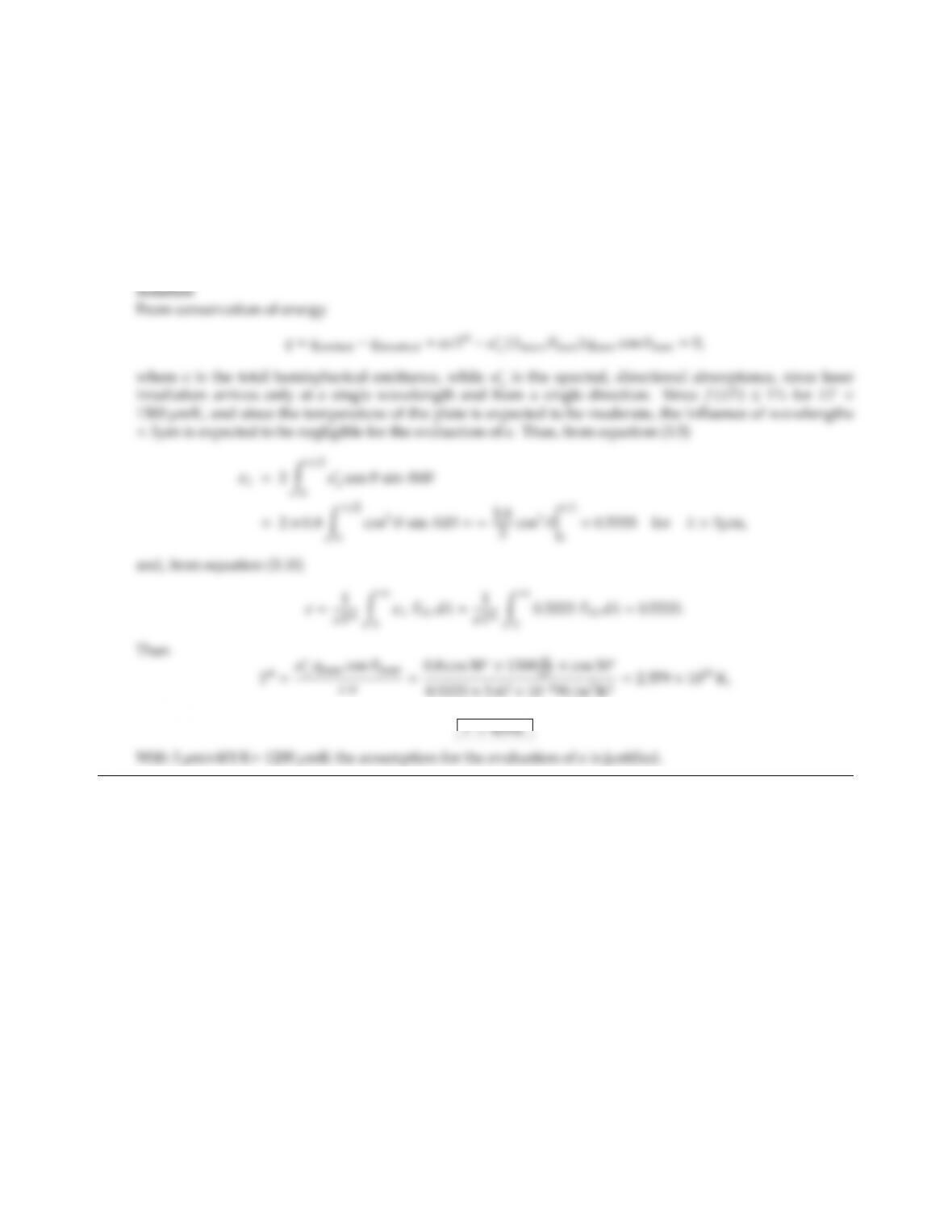CHAPTER 3 45
3.18 A small plate, insulated at the bottom, is heated by irradiation from a defocussed CO2laser beam (wavelength
10.6 µm) with an incidence angle of 30o-normal. The radiative properties of the surface are
ǫ
λ=(0.2 cos2θ, λ < 3µm,
0.8 cos θ, λ > 3µm.
The strength of the laser beam is 1300 W/m2. Neglecting losses due to natural convection, determine the
temperature of the plate.
Note: for such weak laser irradiation levels the heating eect is relatively small.
or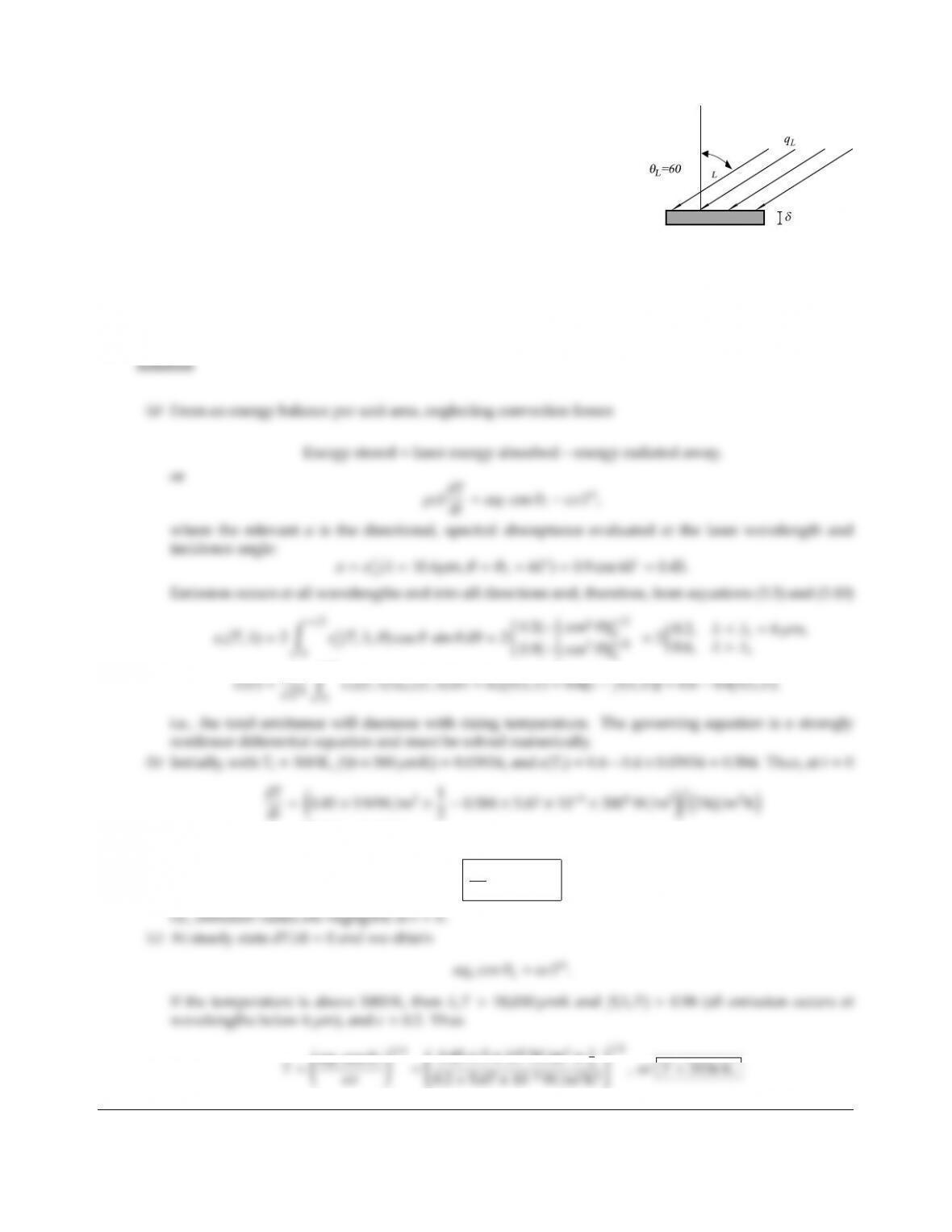3.19
θ
°
A thin disk, insulated at the bottom, is irradiated by a CO2laser (λ=
10.6µm) as shown. The top surface is exposed to a low temperature
(300K) environment. Assuming that the entire disk surface is uniformly
irradiated with qL=5 MW/m2and that the specific heat/area of disk is
ρcpδ=2 kJ/m2K. The disk is at ambient temperature when the laser is
turned on. The emittance of the disk surface is
ǫ
λ=0.2, λ < 6µm,
0.9 cos θ, λ > 6µm.
(a) Indicate how to calculate the temperature history of the disk.
(b) Determine the initial heating rate (in K/s) at t=0.
(c) What is the steady state temperature of the disk? (this is expected to be very high, say >3000 K).
0
3cos3θ)|π/2
0
=(562.50.13) K/s,
dT
dt =562 K/s
0.45 ×5×106W/m2×1

## Trusted by Thousands ofStudents

Here are what students say about us.